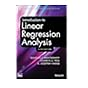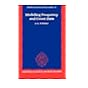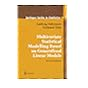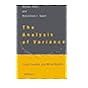Normal view

# Correlated data analysis : modeling, analytics, and applications / Peter X.-K. Song.

Material type:TextLanguage: English Publisher: New York : Springer, c2007Description: xv, 346 p. : ill. ; 24 cmISBN: 9780387713922 (acidfree paper); 0387713921 (acidfree paper); 9780387713939 (e-ISBN)DDC classification: 519.537 LOC classification: QA278.2 | .S615 2007Online resources: WorldCat details | E-book Fulltext
Contents:
Table of contents 1. Introduction and examples -- 2. Dispersion models -- 3. Inference functions -- 4. Modeling correlated data -- 5. Marginal generalized linear models -- 6. Vector generalized linear models -- 7. Mixed-effects models: likelihood-based inference -- 8. Mixed-effects models: Bayesian inference -- 9. Linear predictors -- 10. Generalized state space models -- 11. Generalized state space models for longitudinal binomial data -- 12. Generalized state space models for longitudinal count data -- 13. Missing data in longitudinal studie
Summary: Summary: This book covers recent developments in correlated data analysis, using the class of dispersion models as marginal components in the formulation of joint models for correlated data. Much new material Read more...
Tags from this library: No tags from this library for this title.Average rating: 0.0 (0 votes)
Item type Current location Collection Call number Copy number Status Date due Barcode Item holdsE-Book
E-book
Non-fiction 519.537 SOC 2007 (Browse shelf) Not for loanText
Reserve Section
Non-fiction 519.537 SOC 2007 (Browse shelf) C-1 Not For Loan 26630Text
Reserve Section
Non-fiction 519.537 SOC 2007 (Browse shelf) C-2 Not For Loan 26890Text
Circulation Section
Non-fiction 519.537 SOC 2007 (Browse shelf) C-3 Available 26891
Total holds: 0
##### Browsing EWU Library shelves, Shelving location: E-book Close shelf browser519.536 INT 2012 Introduction to linear regression analysis / 519.536 LIM 1995 Modelling frequency and count data / 519.536 MES 2012 A second course in statistics : 519.537 SOC 2007 Correlated data analysis : 519.538 FAM 2001 Multivariate statistical modelling based on generalized linear models / 519.538 FRE 2011 Exploratory multivariate analysis by example using R / 519.538 SAA 2000 The analysis of variance :

Includes bibliographical references and index.

Table of contents 1. Introduction and examples --
2. Dispersion models --
3. Inference functions --
4. Modeling correlated data --
5. Marginal generalized linear models --
6. Vector generalized linear models --
7. Mixed-effects models: likelihood-based inference --
8. Mixed-effects models: Bayesian inference --
9. Linear predictors --
10. Generalized state space models --
11. Generalized state space models for longitudinal binomial data --
12. Generalized state space models for longitudinal count data --
13. Missing data in longitudinal studie

Summary:
This book covers recent developments in correlated data analysis, using the class of dispersion models as marginal components in the formulation of joint models for correlated data. Much new material Read more...

Applied Statistics

There are no comments for this item.

to post a comment.
Share

Library Home | Contacts | E-journals
Copyright @ 2011-2019 EWU Library
East West University